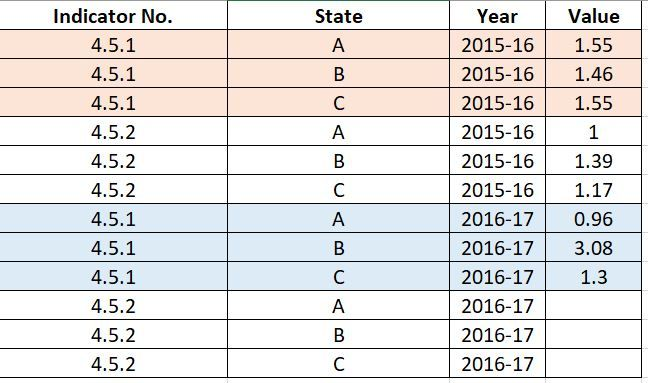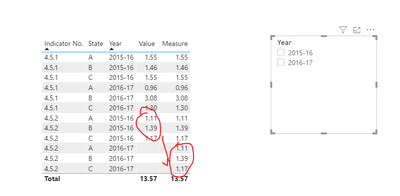cancel
Showing results for
Did you mean:Helper II

## Need help to calculate measure that uses latest non-missing values

Dear Community Members

Apologies for multiple posts related to this topic.

Please help me in solving a problem that looks simple, but I am stuck with it. I am calculating a measure (a score) using the values of indicators for my states. My report has a slicer where users will choose a year to get the score for that particular year. In my data, year is entered as text in the form of: 2011-12, 2015-16, 2016-17, etc.

The measure works well as long as we have data for the indicator in the selected year. If the indicator values are missing for the selected year, my measure is missing for that year too. What I want is that my measure should include the most recent value of the indicator in case the indicator is missing for the selected year. For instance, if user selects 2016-17 the indicator has values only till 2015-16, then the measure should consider the value for 2015-16 to calculate the score.  Following is the DAX code for my measure (sample data are attached).

I am not able to include additional DAX commands in following codes to check for whether the values of the indicators are missing for the selected year. If yes, then the measure should calculate the variables (x and minvalue) based on latest non-missing values.

Test measure =
VAR x =
CALCULATE (
MAX ( Data[Value] ),
FILTER (
ALLEXCEPT ( Data, Data[State], Data[Year] ),
Data[Indicator No.] = "4.5.2"
)
)
VAR minvalue =
CALCULATE (
MIN ( Data[Value] ),
FILTER ( ALLEXCEPT ( Data, Data[Year] ), Data[Indicator No.] = "4.5.2" )
)
VAR num = ( x - minvalue )
VAR denom = ( 1 - minvalue )
RETURN
DIVIDE ( num, denom )1 ACCEPTED SOLUTIONCommunity Champion

Your example resulted in a divide by 0 so i changed the "1" in your sample data to a "1.11".

Take a look at the attached file.

I've added a numeric column that refers to year that you can use to refer to the most recent year with a score.

Let me know if you have any questions!

/ J

4 REPLIES 4Community Support

Hi @rammishra ,

Like this?

``````Measure =
VAR x = SUM(Data[Value])
RETURN
IF(
x = BLANK(),
CALCULATE(
SUM(Data[Value]),
FILTER(
ALL(Data),
Data[Indicator No.] = MAX(Data[Indicator No.]) && Data[YearNum] = MAX(Data[YearNum]) -1 && Data[State] = MAX(Data[State])
)
),
SUM(Data[Value])
)``````Best regards,
Lionel Chen

If this post helps, then please consider Accept it as the solution to help the other members find it more quickly.Super User

@rammishra , if not resolved with the last update.Can you share sample data and sample output in table format?Community Champion

Your example resulted in a divide by 0 so i changed the "1" in your sample data to a "1.11".

Take a look at the attached file.

I've added a numeric column that refers to year that you can use to refer to the most recent year with a score.

Let me know if you have any questions!

/ JHelper II

Thanks. This was really helpful. I only had to edit it by replacing "All" by "AllExcept" function. This is because, use of "All" resulted in same value of the measures across states.

Thank you, once again for your suggestions. This was the solution, I was looking for.

Cheers,

RamAnnouncements#### Exclusive opportunity for Women!

Join us for a free, hands-on Microsoft workshop led by women trainers for women where you will learn how to build a Dashboard in a Day!#### Power Platform Conference-Power BI and Fabric Sessions

Join us Oct 1 - 6 in Las Vegas for the Microsoft Power Platform Conference.Top Solution Authors
Top Kudoed Authors
Users online (1,599)